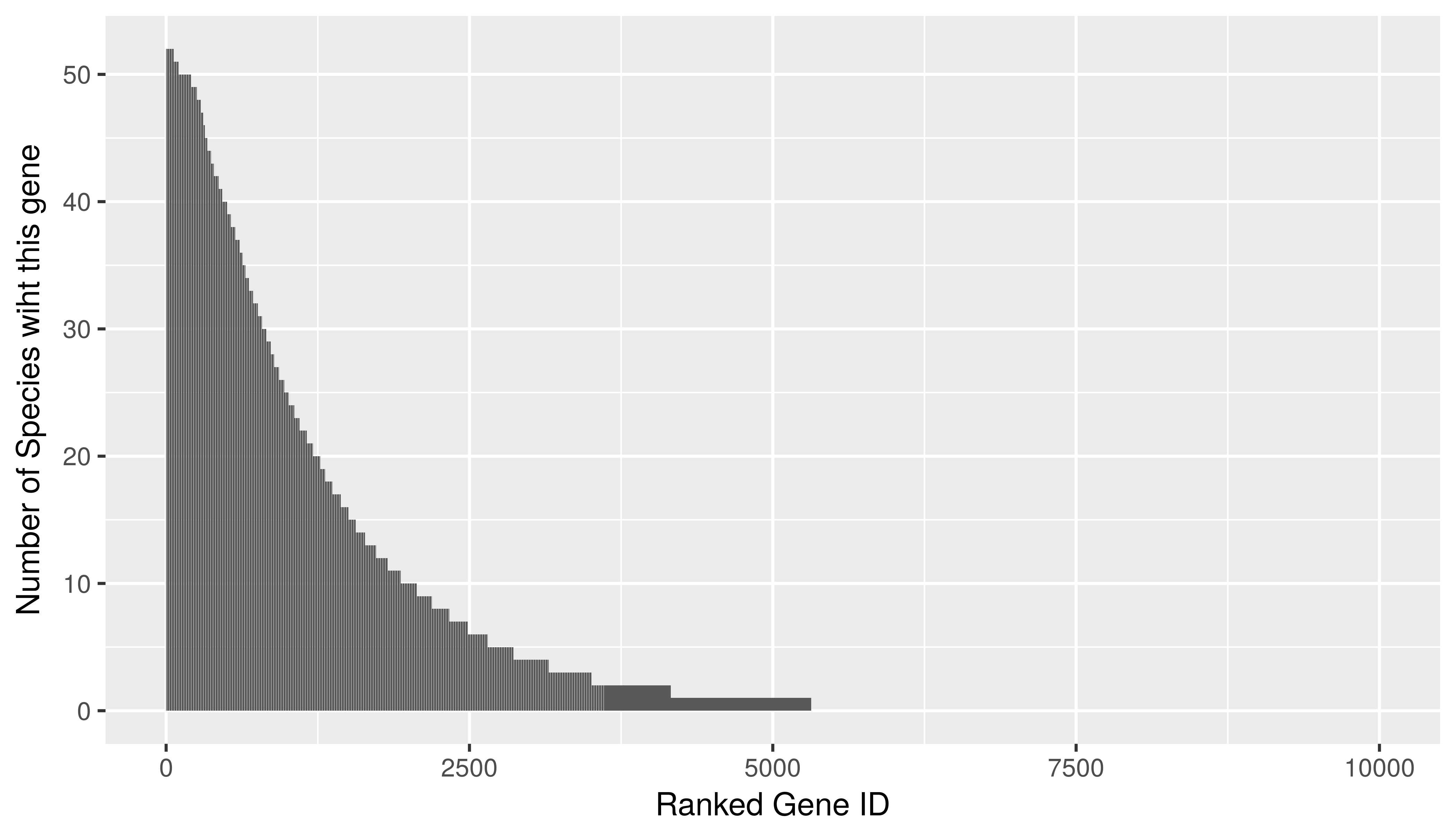$$\newcommand{\bm}{\boldsymbol{\mathbf{#1}}}$$

## Ranked Genes

ranked_gene <- read_delim("ranked_gene.txt",
"\t", escape_double = FALSE, trim_ws = TRUE)

ggplot(ranked_gene, aes(x=rank, y=num_of_species))+
geom_bar(stat = "identity")+
labs(x="Ranked Gene ID",
y="Number of Species wiht this gene")## Random Community

random_community <- read_delim("random_community.txt",
"\t", escape_double = FALSE, col_names = FALSE,
trim_ws = TRUE)[,-53] %>%
set_names(1:52)

df <- gather(random_community, key="Number of Species", value = "Number of genes",
paste(1:52)) %>%
mutate(Number of Species = Number of Species %>% as.numeric()) %>%
filter(Number of genes != 0)

ggplot(df)+
geom_point(aes(x=Number of Species,
y=Number of genes),
size=0.5)+
labs(y="Number of Genes")